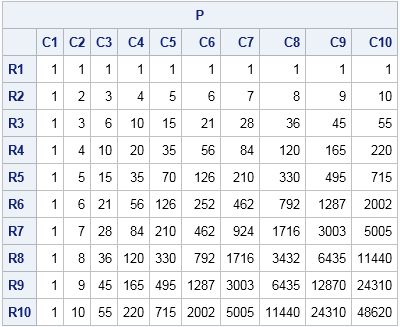A colleague asked me a question regarding my recent post about the Pascal triangle matrix. While responding to his question, I discovered a program that I had written in 1999 that computed with a Pascal triangle matrix. Wow, I've been computing with Pascal's triangle for 15 years! I don't know whether to be proud or embarrassed.

Anyway, here is a neat result from my 1999 program. The Pascal triangle matrix, M, from my last post was lower triangular. Therefore its transpose M` is the Cholesky root of the symmetric positive definite matrix MM`. What is the matrix MM`? The following SAS/IML program computes the answer for a Pascal matrix with 10 rows:

```proc iml; start PascalRule(n); m = j(n,n,0); /* initialize with zeros */ m[,1] = 1; /* set first column to 1 */ j = 2:n; /* elements to compute */ do k = 2 to n; /* for kth row, add adjacent elements from previous row */ m[k,j] = m[k-1,j-1] + m[k-1,j]; end; return(m); finish;   M = PascalRule(10); /* the Cholesky root of some matrix */ P = M * M`; /* symmetric positive definite (SPD) */ print P[F=5. r=("R1":"R10") c=("C1":"C10")];```Tilt your head to the left and look carefully at the antidiagonals of the matrix P. The kth antidiagonal contains values from the kth row of Pascal's triangle! In other words, the Cholesky root of a Pascal matrix contains another Pascal matrix!

I didn't discover this fact. In my 1999 program I have a comment that says

See N. Higham, Accuracy and Stability of Numerical Algorithms, SIAM, 1996, for interesting facts related to the Pascal matrices. In particular, the Cholesky factor of a Pascal matrix has columns that contains the elements of Pascal's triangle!

Several readers posted comments to my previous blog that describe other features of Pascal's matrix. In particular, the inverse and square of a Pascal matrix exhibit interesting characteristics.

By the way, my program from 1999 actually started with the symmetric positive definite Pascal matrix and computed the triangular matrix by calling the ROOT function in SAS/IML. The following module computes the symmetric positive definite Pascal matrix directly:

```/* compute the SPD matrix whose antidiagonals are the rows of Pascal's triangle */ start PascalMatrix(n); P = j(n,n); do i = 2 to n; j = i:n; P[i,j] = comb( i+j-2, j-1 ); P[j,i] = T(P[i,j]); /* make symmetric */ end; return ( P ); finish;```
ShareDistinguished Researcher in Computational Statistics

Rick Wicklin, PhD, is a distinguished researcher in computational statistics at SAS and is a principal developer of SAS/IML software. His areas of expertise include computational statistics, simulation, statistical graphics, and modern methods in statistical data analysis. Rick is author of the books Statistical Programming with SAS/IML Software and Simulating Data with SAS.

1.Did you know that the Pascal Triangle Matrix has a square root, which is formed from itself scaled by the powers of two?
.
a = PascalRule(10);
b = a # 2 ## (col(a) - row(a));
c = b * b;
print a, b, c;
.
See Barry Lewis (2008), "More Power to Pascal", The Mathematical Gazette, v92(525), pp454-465.

•Fascinating!

2.can you please provide a solution for pascal triangle using backtracking...